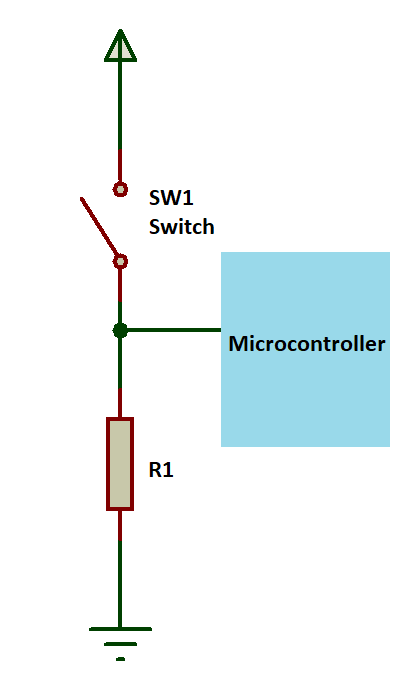# PULL UP RESISTOR AND PULL DOWN RESISTOR

Pull UP resistor and Pull Down resistor are the most important concepts in electronic circuits.

NOTE: Pull down – PD & Pull up – PU

## PULL UP RESISTOR

Pull up resistors are simple fixed value resistors, which are connected between the voltage supply and the particular I/O pin.

Pull up resistors are used in digital logic circuits to make sure logic level or logic state at a pin is HIGH. Digital logic circuits consist of three states LOW, HIGH and floating or high impedance.

When you pass 5V at a microcontroller pin it is considered as HIGH and when you pass 0V or GND as input considered as LOW.

When the microcontroller pin is not pulled to any HIGH or LOW logic level, then the floating or high impedance state occurs. To avoid this high impedance state these resistors are used by pulling it to a high state, as seen in the figure.

Using a pull up resistor we want input to remain high in the absence of an input signal. Because when we press the button its state will change and when we release the button its state should become the same as the previous state (HIGH) not to be floating.

Generally, we use a PU resistor value of about 4.7 KΩ, but can change depending on the application.

### CIRCUIT DIAGRAM##### FOR EXAMPLE

When you try to read LOW logic using a microcontroller, You need to connect a button and ground in series.

As you press the button the state of the microcontroller pin will become LOW, but when you release the button, pin state will not change (floating or high impedance state) then you need to give some logic to change state. Then we use a Pull up resistor which sets the microcontroller pin to HIGH state.

## PULL DOWN RESISTOR

Pull up & pull down resistors are similar. But, pull down resistors, pull the pin to a low value (opposite to PU).

Pull down resistors are also fixed value resistors, which are connected between a particular pin (generally input) on a microcontroller and the ground terminal. Digital circuit shown in the figure below is an example of a PD resistor.

Using the Pull Down resistor we want input to remain LOW in the absence of an input signal. Because when we press the button its state will change and when we release the button its state should become the same as the previous state (LOW) not to be floating.

Generally, we use a PD resistor value of about 10 KΩ, but can change depending on the application.

### CIRCUIT DIAGRAM##### FOR EXAMPLE

When you try to read HIGH logic using a microcontroller, You need to connect a button and power (+5V) in series.

As you press button the state of microcontroller pin will become HIGH, but when you release button, pin state will not change (floating or high impedance state) then you need to give some logic to change state. Then we use PD resistor which sets the microcontroller pin to LOW state.

The PD resistor should have a higher resistance than the input impedance of the logic circuit.

NEXT POST
TRANSISTOR – BJT BC547

PREVIOUS POST
LED – LIGHT EMITTING DIODE